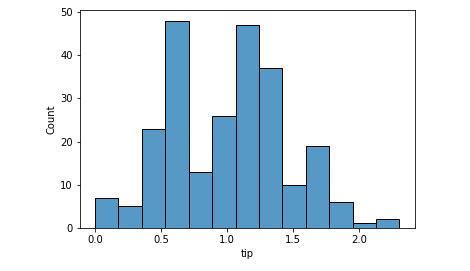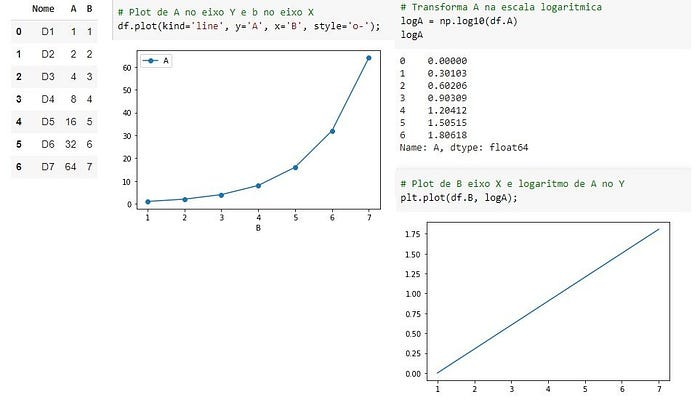# Normalization, Standardization and Normal Distribution

Understand the difference, when to use and how to code it in Python

I will start this post with a statement: normalization and standardization will not change the distribution of your data. In other words, if your variable is not normally distributed, it won’t be turn into one with the `normalize` method.

`normalize()` or `StandardScaler()` from `sklearn` won’t change the shape of your data.

## Standardization

Standardization can be done using `sklearn.preprocessing.StandardScaler` module. What it does to your variable is centering the data to a mean of 0 and standard deviation of 1.

Doing that is important to put your data in the same scale. Sometimes you’re working with many variables of different scales. For example, let’s say you’re working on a linear regression project that has variables like years of study and salary.

Do you agree with me that years of study will float somewhere between 1 to 30? And do you also agree that the salary variable will be within the tens of thousands range?

Well, that’s a big difference between variables. That said, once the linear regression algorithm will calculate the coefficients, naturally it will give a higher number to salary in opposition to years of study. But we know we don’t want the model to make that differentiation, so we can standardize the data to put them in the same scale.

`import pandas as pdimport seaborn as snsfrom sklearn.preprocessing import StandardScaler, normalizeimport scipy.stats as scs# Pull a datasetdf = sns.load_dataset('tips')# Histogram of tip variablesns.histoplot(data=df, x='tip');`

Ok. Applying standardization.

`# standardizingscaler = StandardScaler()scaled = scaler.fit_transform(df[['tip']])# Mean and Std of standardized dataprint(f'Mean: {scaled.mean().round()} | Std: {scaled.std().round()}')[OUT]: Mean: 0.0 | Std: 1.0# Histplotsns.histplot(scaled);`

The shape is the same. It wasn’t normal before. It’s not normal now. And we can take a Shapiro test for normal distributions before and after to confirm. The p-Value is the second number in the parenthesis (statistic test number, p-Value) and if smaller than 0.05, it means not normal distribution.

`# Normal test original datascs.shapiro(df.tip)[OUT]: (0.897811233997345, 8.20057563521992e-12)# Normal test scaled datascs.shapiro(scaled)[OUT]: (0.8978115916252136, 8.201060490431455e-12)`

## Normalization

Normalization can be performed in Python with `normalize()` from `sklearn` and it won’t change the shape of your data as well. It brings the data to the same scale as well, but the main difference here is that it will present numbers between 0 and 1 (but it won’t center the data on mean 0 and std =1).

One of the most common ways to normalize is the Min Max normalization, that basically makes the maximum value equals 1 and the minimum equals 0. Everything in between will be a percentage of that, or a number between 0 and 1. However, in this example we’re using the normalize function from sklearn.

`# normalizenormalized = normalize(df[['tip']], axis=0)# Normalized, but NOT Normal distribution. p-Value < 0.05scs.shapiro(normalized)[OUT]: (0.897811233997345, 8.20057563521992e-12)`

Again, our shape remains the same. The data is still not normally distributed.

## Then why to perform those operations?

Standardization and Normalization are important to put all of the features in the same scale.

Algorithms like linear regression are called deterministic and what they do is to find the best numbers to solve a mathematical equation, better said, a linear equation if we’re talking about linear regression.

So the model will test many values to put as each variable’s coefficients. The numbers will be proportional to the magnitude of the variables. That said, we can understand that variables floating on the tens of thousands will have higher coefficients than those in the units range. The importance given to each will follow.

Including very large and very small numbers in a regression can lead to computational problems. When you normalize or standardize, you mitigate the problem.

## Changing the Shape of the Data

There is a transformation that can change the shape of your data and make it to approximate of a normal distribution. That is the logarithmic transformation.

`# Log transform and Normality scs.shapiro(df.tip.apply(np.log))[OUT]: (0.9888471961021423, 0.05621703341603279)p-Value > 0.05 : Data is normal# Histogram after Log transformationsns.histplot(df.tip.apply(np.log) );`Variable ‘tip’ log transformed. Now it is a normal distribution. Image by the author.

The log transformation will remove the skewness of a dataset because it puts everything in perspective. The variances will be proportional rather than absolute, thus the shape changes and resembles a normal distribution.

A nice description I saw about this is that log transformation is like looking at a map with a scale legend where 1 cm = 1 km. We put the whole mapped space on the perspective of centimeters. We normalized the data.

## When to Use Each

As far as I researched, there is no consensus whether it’s better to use Normalization or Standardization. I guess each dataset will react differently to the transformations. It is a matter of testing and comparing, given the computational power these days.

Regarding the log transformation, well, if your data is not originally normally distributed, it won’t be a log transformation that will make it that way. You can transform it, but you must reverse it later to get the real number as prediction result, for example.

The Ordinary Least Squares (OLS) regression method — calculates the linear equation that best fits to the data considering that the sum of the squares of the errors is minimum — is a math expression that predicts y based on a constant (intercept value) plus a coefficient multiplying X plus an error component (y = a + bx + e). The OLS method operates better when those errors are normally distributed, and the analyzing the residuals (predicted – actual value) are the best proxy for that.

When the residuals don’t follow a normal distribution, it is recommended that we transform the independent variable (target) to a normal distribution using a log transformation (or another Box-Cox power transformation). If that is not enough, then you can try transforming the dependent variables as well, aiming for a better fit of the model.

Thus, log transformation is recommended if you’re working with a linear model and needs to improve the linear relationship between two variables. Sometimes the relationship between variables can be exponential and log is the inverse operation of the exponential power, thus a curve becomes a line after transformation.An exponential relationship that became a line after a log transformation. Image by the author.

## Before You Go

I am no statistician or mathematician. I always make that clear and I also encourage statisticians to help me to explain this content to a broader public, the easiest way possible.

It is not easy to explain such a dense content in simple words.

I will end here with these references.

Why to log transform.

Normalization and data shape.

Normalize or Not.

When to Normalize or Standardize.Data Scientist. I extract insights from data to help people and companies to make better and data driven decisions.